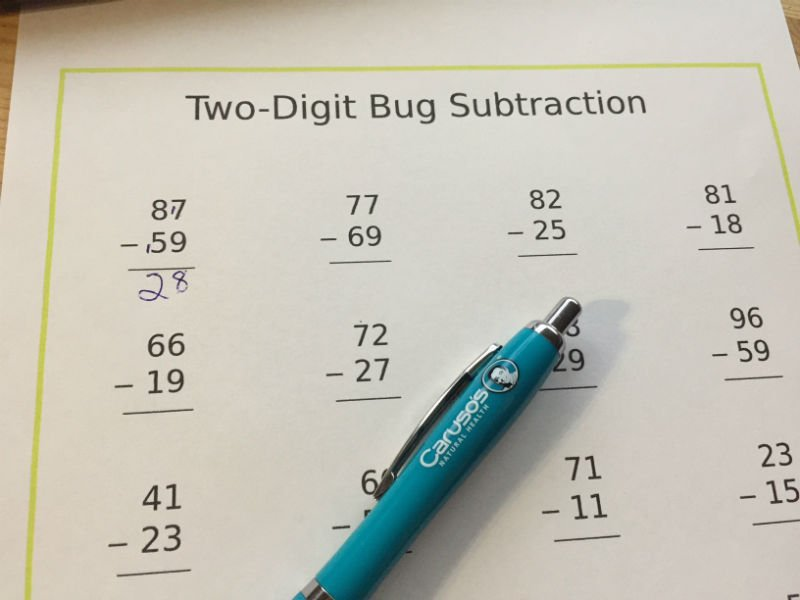My kids seem very happy when they are adding things together, but things get complicated very quickly when they have to subtract numbers from each other. You can see the stress and worry on their faces.

As soon as things start to get more complicated the girls seem to panic.

My technique is to be calm, to concentrate on the question being asked, and to work out little bits at a time.I like many parents have shown the girls how to do various sums, and told them that it is just a matter of a little practice each day. In no time they will get better and be a champion at maths.

The one thing that I believe is not being understood is the carrying the numbers over or what I was told was carrying the one or another number into the other column.The way I figure out subtraction, and the way I am showing the girls. Carrying the 1 or what is called borrowing in subtraction.

As you can see from the above example of 87 – 59. You cannot have 7 taking away from 9.

So to make it easier, you add a 1 to 7 and make it 17.

Since you have added the 1 to 7 to make it 17, you then need to carry the 1 to the tens column.

I add the 1 to the 5 and this then becomes 6. So then it will be 8 -6 and this gives you 2 in the tens column, and as it is now 17 – 9 in the ones column this means you get 8, and therefore the answer is 28.

This following video can explain it more easily to kids and explains the idea of borrowing or like I was taught carrying the one.

### HOW TO BORROW IN SUBTRACTION FOR KIDS

/center>

So what can you do to promote practice in subtraction or really any maths problem?

Education.com have some great resources that will help your kids practice and also have fun doing so.

Crawl around these subtraction problems with the cute creepy crawlies in this worksheet! Can’t get enough subtraction practice? Check out the full collection of subtraction resources from Education.com!